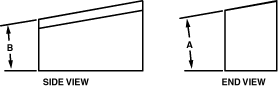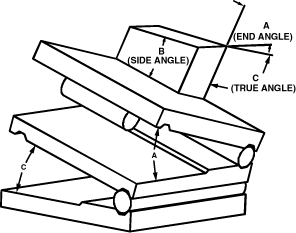>

 COMPOUND ANGLE COMPENSATIONWhen setting compound angles, it is necessary to compensate for the first angle set in order to correctly set the second angle. To obtain this compensation, refer to the following drawings and procedures. where: Angle A = First desired angle Angle B = Second desired angle Angle C = True angle setting required to get angle B VIEW OF PART Set the first of the desired angles (Angle A) on the top section of your compound sine plate. Calculate the tangent of the True Angle (Angle C) using the formula:Tan (True Angle C) =Tan (Angle B) X Cos (Angle A) Lookup the True Angle (Angle C) in a table of tangents (or use the inverse tangent function on your calculator).Set the lower section of your compound sine plate, using the proper sine table and the True Angle (Angle C). Results: The compound sine plate is now set to obtain the desired angles (Angles A & B) in their respective planes.

Automation and Metrology 130 Liberty Street Painesville, Ohio 44077
Phone:  (440) 354 6436  Fax:  (440) 639 9983
E-mail: sales@auto-met.com

www.auto-met.com